﻿ The Relationship between Eighth Grade Elementary Students’ Operational and Measurable Prediction Skills and Mathematical Literacy

### The Relationship between Eighth Grade Elementary Students’ Operational and Measurable Prediction Ski...

Ömer Faruk Çetin, Kemal KöseOPEN ACCESSPEER-REVIEWED

## The Relationship between Eighth Grade Elementary Students’ Operational and Measurable Prediction Skills and Mathematical Literacy

Ömer Faruk Çetin1, Kemal Köse2

1Elementary Mathematics Education Department, Erzincan University, Erzincan, TURKEY

2Ministry of National Education, Ordu, TURKEY

### Abstract

This study aimed to investigate relationship between eighth grade elementary students’ operational and measurable prediction skills and their mathematical literacy. Mathematical literacy is a very broad term but in the present study it was narrowed with students’ daily life issues and used for regarding students’ mathematics usage in their daily life according to their levels. In the study, quantitative methodology to investigate the levels of the students’ prediction skills and mathematical literacy and qualitative methodology to determine the students’ strategies in the prediction question were chosen. The participants of the study were chosen with a stratified sampling random strategy from a medium-scaled city of East Anatolian Region of Turkey in the academic year 2011–2012. The participants were 221 eighth grade students from 20 national elementary schools. As data collection tools, a “Measurable and Operational Prediction Skill Test” including ten questions for the prediction skill and “Mathematical Literacy Test” including 20 questions were used. The findings showed that there was a significant relationship between measurable and operational prediction skills. Also it was observed that the students having high score of measurable and operational prediction skills became more successful in the mathematical literacy test. The gender did not have any significant impact on these variables.

• Çetin, Ömer Faruk, and Kemal Köse. "The Relationship between Eighth Grade Elementary Students’ Operational and Measurable Prediction Skills and Mathematical Literacy." American Journal of Educational Research 3.2 (2015): 142-151.
• Çetin, Ö. F. , & Köse, K. (2015). The Relationship between Eighth Grade Elementary Students’ Operational and Measurable Prediction Skills and Mathematical Literacy. American Journal of Educational Research, 3(2), 142-151.
• Çetin, Ömer Faruk, and Kemal Köse. "The Relationship between Eighth Grade Elementary Students’ Operational and Measurable Prediction Skills and Mathematical Literacy." American Journal of Educational Research 3, no. 2 (2015): 142-151.

 Import into BibTeX Import into EndNote Import into RefMan Import into RefWorks

### 1. Introduction

Mathematics is a natural effort of the human being who makes some generalizations from the concrete objects to abstract events and tries to reach world-wide general acceptance of these generalizations with a systematic doctrine. This effort progresses each day and gradually gains importance. Therefore, all countries give some place to teaching mathematics in their educational curricula. Mathematics education is much more than teaching numbers, mathematical operations, calculation skills which are the indispensable parts of daily life, it is actually related to teaching thinking, making connections between events, reasoning, making predictions and problem-solving . One of the fundamental goals of mathematics education is using mathematics as a tool that facilities our life. Therefore, in order to overcome barriers faced in daily life students need some skills such as critical thinking, reasoning, evaluating the cases and generating predicting for a present issue. The prediction skill, one of these skills is one of the fundamental skills that facilitate our life . Predicting a part or total of a problem is not a random event, it is highly dependent on an individual’s mathematical knowledge. Then, elementary mathematics curricula give place to the prediction skill . Predictions can be right or wrong, and the issue can be resulted in an expected way or not. In that case, the result does not matter much as it develops an individual’s many mathematical skills such as logical relations, spatial visualizations, understanding measurements and numbers ([6, 20]). Although the prediction is defined differently in many studies (e.g. [23, 25, 27, 33]), it is probable to say that it is a kind of calculation reaching a probable result without using paper and pen ([2, 9]). The prediction used in many areas is divided into three types as cumulative prediction ([2, 16, 38, 41]), measurable prediction ([2, 41]) and operational prediction ([2, 9, 17, 25, 26, 29, 38, 41]). Operational prediction is the processes of getting approximate arithmetic mean scores without calculation ([2, 9, 17, 26, 38]). Operational prediction is a complex skill including problem-solving features . One of the strongest and beneficial ways of operational prediction is its facilitating role of the life with the usage in daily life. With the prediction, one can measure an ordinary event, daily life issues and mathematical experiences . Measurable prediction is a kind of prediction generally used for predicting length, weight, liquid capacity or similar measurements. It also includes predicting the weight of a tool or a pen, the height of a building, the length of a rope or the perimeter of a area . With the effects of the studies about the prediction skills (e.g., [2, 3, 4, 5, 8, 10, 11, 14, 15, 17, 21, 23, 27, 28, 30, 33, 34, 35, 38, 40, 41, 43]), teaching mathematics curricula are developed and modified according the actual needs. In order to be updated to reach these developments, Turkey has made radical changes in teaching mathematics curricula of elementary schools and applied new programs after 2005 in Turkey. Then, new topics such as “patterns, transformation geometry, probability, object graph and prediction” were added into the curricula. As the concepts in mathematics like prediction skill, knowledge of formula and operations are necessary for all citizens, all individuals need some literacy on these issues and mathematical knowledge ([12, 13]). Relating life with the mathematics in the school or developing simply students’ abstract thinking skills is not an adequate for the goal of a universal discipline, mathematics . Mathematical literacy ([1, 13, 18, 24, 32, 37, 39]) is much more than doing mathematical operations and in reality it is the level of determining mathematical problems in the context of real life, defining them as mathematical problems and overcoming these problems ([31, 37]). Individuals equipped with the knowledge of mathematical literacy have the skill of determining and using the mathematical relations among social, updated and scientific events. Also those are conscious citizens and consumers analysing and interpreting the knowledge in newspapers, TV channels and internet . They can decide on the accuracy and logicalness of the probable results of a problem. In a limited time or less time to calculate with measurement tools, they can use their prediction skills in order to predict the accurate solution . Therefore, there is a need for the investigation of individuals’ prediction skills and mathematical literacy.

The aim of this study is to investigate the relationship between eighth grade elementary students’ operational and measurable prediction skills and mathematical literacy. Below sub-problems related to this aim were asked in the study.

1. Is there a relationship between the levels of students’ operational and measurable prediction skills?

2. Do the students’ levels of prediction differ with regards to their gender?

3. Do the students’ levels of mathematical literacy differ with regards to their sex?

4. How do the students’ skills related to the calculation of length, area and volume in the test of mathematical literacy?

5. Do the students’ prediction skills differ with regards to their mathematical literacy?

### 2. Method

The survey research which aims to determine a high numbers of participants’ ideas, features  was used in the study. In a survey research, the whole population or a part of the population are chosen for gathering a general conclusion about the population . In description, it is aimed to describe the given issue exactly and in detailed way . In a quantitative research, the precise limits of the variables can be determined and the relationships between the variables can be measured. The causation in the quantitative research can be dealt in a neutral and objective way (Glesne and Peksin, 1992 cited in ).

2.1. The Participants of the Study

The participants of the study were comprised of 221 eighth grade students from 20 elementary schools located in East Anatolian Region of Turkey. 112 students were female and 109 students were male students in the study.

2.2. Data Collection Tools and Data Collection Method

The data in the study were collected with a “Prediction Skill Test (PST)” which has 10 open-ended questions having five measurable and five operational prediction skill questions, and a “Mathematical Literacy Test (MLT)” which has 20 questions related to length, area and volume. The details about the data collection tools were given below.

The PST was adapted from the studies of [27, 28, 30]. Below steps were applied in the process of prediction skill test development.

1. After being analysed the sixth, seventh and eighth grade mathematics education curricula, the outcomes related to the prediction in textbooks were determined.

2. Based on the analysed outcomes, the prediction questions from the texts books about the topics of length, area and volume were prepared.

3. In order to support the face and content validity, the test was reviewed by three mathematics teachers and their ideas were taken. Based on their ideas, some questions were modified or removed from the test. Later, two experts’ ideas about the test were taken and some corrections based on the experts were done. Lastly, two Turkish language teachers’ ideas about the proper language use in the test were taken.

4. The test was given to 24 students and they were informed about the aim of test application and the study. During the application it was observed that test was finished within 20 minutes. During the application, the students’ questions were not answered there and they were noted. Later, they were asked to give details about the missing points in the test. As it was observed that the students did not answer sixth and ninth questions and lived some difficulties in understanding them. Therefore, these questions were changed the new ones.

5. After necessary revisions, the new test was re-applied to 20 students and the students did not asked any questions. Based on the last application, the reliability analyses were conducted.

6. After the second pilot study with 20 students, the Cronbach Alpha technique was used for the reliability measurable and operational prediction skill test.

The questions that students lived difficulty in answering and understanding were changed and new ones were used instead of them. These questions were given below.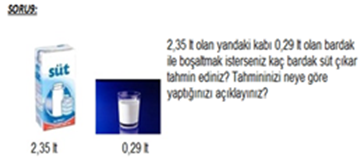Question 9. How many glasses of milk do you gather if you want to empty the 2,35 litre- milk packet with a 0,29 litre-glass? Please explain your prediction.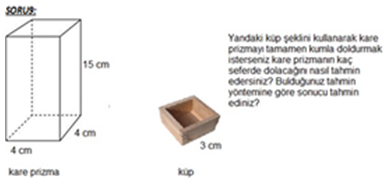Question 9: If you want to fill in the square prism with sand via the cube figure, how do you predict when the square prism is filled? Please, predict the answer according to your way of prediction.

The first question was changed as the graph seemed more complicated for understanding for the students and the second question was changed as the objects there were not suitable for the prediction and the students tried to answer the question with the operation. PST questions were as follow:

Question-1. (Operational)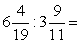If you want to predict the result of this mathematical operation, how do you answer it? Please, predict the answer according to your way of solution.

Question-2. (Measurable)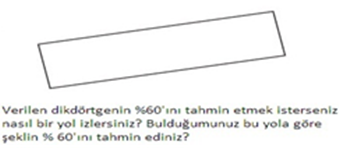If you want to predict 60% of this rectangle, how do you answer it? Please, predict 60% of the figure according to your way of answer.

Question-3. (Operational)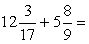If you want to predict the result of this mathematical operation, how do you answer it? Please, predict the answer according to your way of solution.

Question-4. (Measurable)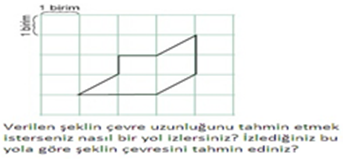If you want to predict peripheral length of the figure, how do you answer it? Please, predict the peripheral length of the figure according to your way of answer?

Question-5. (Operational)

7, 098. 5, 61 =

If you want to predict the result of this mathematical operation, how do you answer it? Please, predict the answer according to your way of solution

Question-6. (Measurable)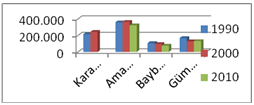Four cities’ population numbers in years 1990, 2000 and 2010 are given but the city, Karaman’s population number in the year 2010 is not given in the graph. If you want to predict the population of Karaman in 2010 according to the graph, how do you answer it

Question-7. (Operational)

24, 708 – 9,006 =

If you want to predict the result of this mathematical operation, how do you answer it? Please, predict the answer according to your way of solution

Question-8. (Measurable)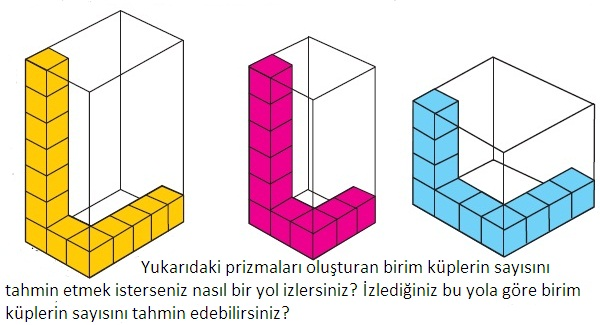If you want to predict the number of unit cubes that unite the above prisms, how do you answer it? Please, predict the number of unit cubes according to your way of answer.

Question-9. (Operational)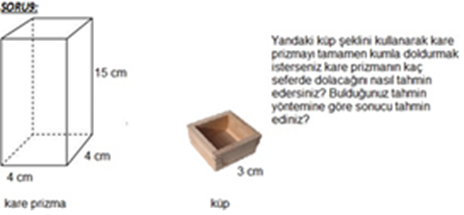If you want to fill in the square prism with sand via the cube figure, how do you predict when the square prism is filled? Please, predict the answer according to your way of prediction.

Question-10. (Measurable)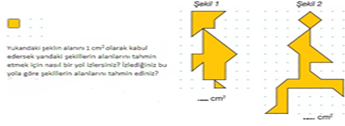If you accept the area of the above figure as 1 cm 2, how do you predict the area of the figures? Please, predict the area of the figures according to your way of prediction.

In a similar way, a literature review was conducted for the development of MLT and the questions related to the students’ daily life were prepared. The test development steps were as follow:

1. Firstly, the students’ daily usage areas of mathematics in life were determined.

2. Later, some categorizations like mathematics in home, game, shopping and daily life were done and the length, area and volume questions about these categories were prepared.

3. The test questions were presented to two mathematics teachers and three experts and some revisions were done according to their suggestions. The test was evaluated by two Turkish language teachers in view of language use, spelling and semantics.

4. After these processes, the test was applied to 24 students and the pilot study lasted in 20 minutes. During the application, the students’ questions were not answered and they were noted. After the application, the students were asked about the missing points of the questions and the reasons of their misunderstanding were taken. Based on the students’ responses, some changes were done.

The questions that students lived difficulty in answering and understanding were changed and new ones were used. These questions were as follow.

Question 1: Think approximately how many m2s can be the area of classroom floor.

Question 1: How many m2s is the area of your classroom floor approximately?

Question 19: Think how many handbreadths can be the peripheral of the tennis table?

Question 19: How many handbreadths is there a table having 200 cm width and 249 cm length?

As the first question’ expression “Think approximately” caused misunderstandings and the second question was not relevant for the prediction and the students could not answer them properly, they were changed with side questions based on the experts’ suggestions. The questions of MLT were as follow:

1. How many cm2s is the area of your classroom floor approximately?2. How many cm3s is the volume of your classroom computer tower approximately

3. How many footsteps do you take steps from the entrance gate of your school to the classroom door

4. How many cm2s is the perimeter of your classroom board approximately?

5. How many students are there in your school approximately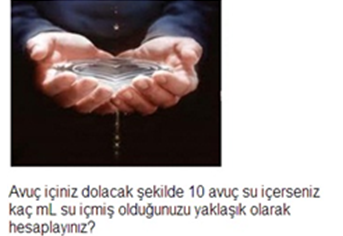6. If you drink water with 10 full of palms, how much ml water do you drink approximately7. How many m2s is the area of LCD TV if the area of wall is 6 m2s in the picture?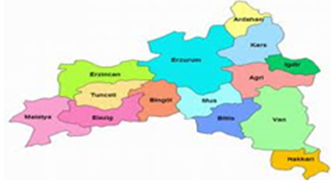8. The area of East Anatolian Region is 164.000 km2s. Then, what is the approximate area of the city of Erzincan?

9. You are living with a new-born sibling, a 19 year-old older brother, 35 year-old mother and 43 year-old father. What is the approximate age mean of your family?10. What is the approximate volume of the backpack?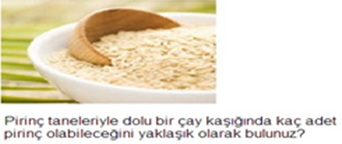11- How many pieces of rice can be approximately in a tea spoon?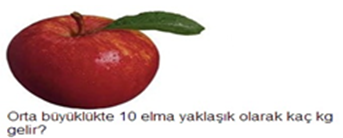12. How many kilograms can be ten medium-sized apples?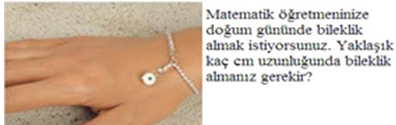13. You want to buy a wristband for your mathematics teacher for her/his birthday. How many centimetres can it be approximately?14. Your 12-year old friend win the picture wants to go the tailor for a suit. How many square meters does the tailor need for that suit?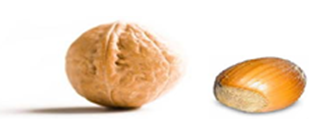15. If an average nut’s volume is 4 cm3s, what is the approximate volume of a walnut?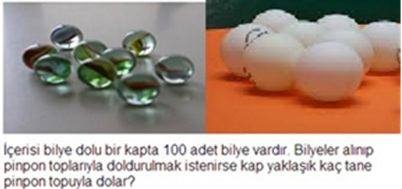16. There are 100 marbles in a container. If you want to fill in the container with ping pong balls instead of marbles, how many balls can you need approximately?

17. You will play a game with ten people with a footstep distance between students by creating a circle. Find the approximate area of the circle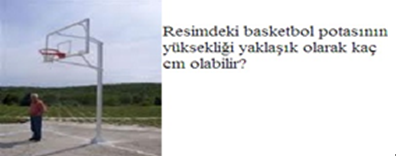18. How many centimetres can be the basketball basket in the picture?

19. How many handbreadths is there a table having 200 cm width and 249 cm length?

20. You will play a game with your friend on the prediction of the numbers of sheets. Let’s see who will find the closest answer. How many sheets are there here?

2.3. Data Collection

In the first step, the permissions from the relevant institutions for the application were taken and the mathematics teachers of the schools were informed about the application. The mathematical literacy test and prediction skill test were applied to students in the class during a course hour period. At the beginning, the students were told about the aim and significance of the study, and it was emphasised the difference of the test that they were acquainted with before. The questions were presented to the students with separate two sheets. While students were answering the prediction test, they were asked to explain their ways of solution. For easing the understanding of the mathematical literacy test, some objects were carried into the class and the students were allowed to touch the objects. Some of the students’ behaviours during the answering the questions were photographed and described in detail. In addition, they were informed about painting of 60% of the figure in the second question of the test. Some photographs taken while the students were answering the test of mathematical literacy were shown below.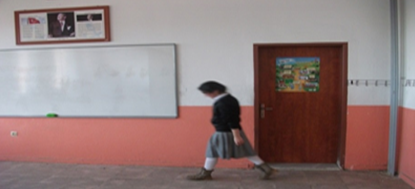The student firstly measured the edges of the class by feet in order to predict the area of the class and then made her prediction based on her step length.In order to calculate the perimeter of the blackboard, the student firstly found how many handbreadths are the edges of the board and then made her prediction based on the prediction of the length of a handbreadth?

2.1.5. Figures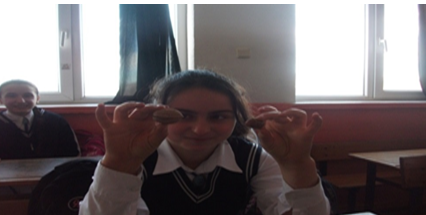She made a prediction on the volume of a nut and a walnut by comparing their volumes.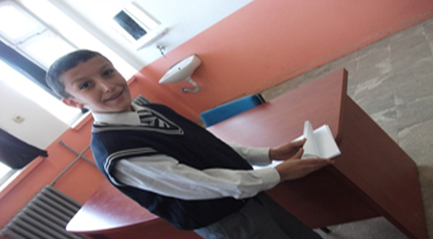He made a prediction by touching the sheets in order to predict the actual numbers of the sheets.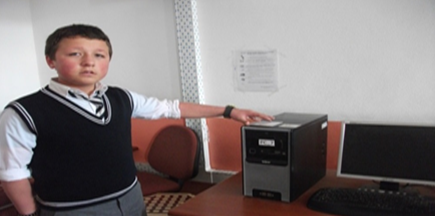He found the volume of a computer tower by calculating the numbers of handbreadths of the tower.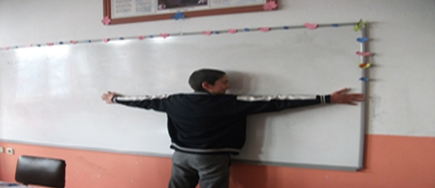He calculated the perimeter of the board with his overarms.

2.4. Data Analysis

The students’ answers to each question were evaluated with a 5-point graduation (i.e. 1, 2, 3, 4 and 5). Each student’s scores of mathematical literacy test, prediction skill test, measurable prediction, operational prediction, area skill, length skill, volume skill were calculated and these scores’ percentages were presented to avoid confusion.

### 3. Findings and Discussions

In this section, the findings about the sub-problems were presented one by one and discussions about the findings were given.

3.1. Findings With Regards to the First Sub-Problem

The findings about Eighth grade students’ levels of operational and measurable prediction skills were presented in Table 1.

#### Table 1. The results of the analysis with regards to the students’ levels of operational and measurable prediction skills

Table 1 showed that there was a positive correlation between the students’ operational and measurable prediction skills (r =0.390).

3.2. Findings With Regards to the Second Sub-Problem

The students’ prediction skills according to their gender type were presented below.

#### Table 2. Prediction skills of students according to gender

When the students’ scores were divided into five categories, it was seen that female students’ and male students’ scores of the prediction skill were close to each other with percentages 41,07% and 44.95%.

The students’ mean, standard deviation and t-test results of the prediction skill with regards to gender were shown in Table 3.

#### Table 3. Mean, standard deviation and t-test results of the prediction skill with regards to gender

According to the Table 3, the female students’ mean of prediction skill was 63,89 and the male students’ mean was 61,66. In spite of this difference, there was not a significant difference between the means in views of gender (t219 :. 859, p >. 05 ).

The students’ mean, standard deviation and t-test results of the operational prediction skill with regards to gender were shown in Table 4.

#### Table 4. Mean, standard deviation and t-test results of the operational prediction skill with regards to gender

According to the Table 4, the female students’ mean of operational prediction skill was 70,07 and the male students’ mean was 67,82. In spite of this difference, there was not a significant difference between the means in views of gender (t219 :. 673, p >. 05 ).

The students’ mean, standard deviation and t-test results of the measurable prediction skill with regards to gender were shown in Table 5.

#### Table 5. Mean, standard deviation and t-test results of the measurable prediction skill with regards to gender

According to the Table 4, the female students’ mean of operational prediction skill was 60,07 and the male students’ mean was 58,20. In spite of this difference, there was not a significant difference between the means in views of gender (t219:. 637, p >. 05 )

3.3. Findings With Regards to the Third Sub-Problem

The students’ scores of mathematical literacy according to their gender type were presented below

#### Table 6. The scores of mathematical literacy of students according to gender

According to the Table 6, it was seen that female students’ (48,22) and male students’ (41,29) scores of the prediction skill were close to each other and their scores are at average level in five categories.

The relationship between the students’ levels of mathematical literacy and gender was presented in Table 7.

#### Table 7. Mean, standard deviation and t-test results of the of mathematical literacy with regards to gender

The Table 7 showed that the female students’ mean of mathematical literacy (63,50%) was less than the male ones’ mean (65,29%). In spite of this mean difference, there was not a significant difference between the means in views of gender (t219 : -1.365, p >. 05 ).

3.4. Findings With Regards to the Fourth Sub-Problem

The means and standard deviations of the students’ skills in the issues of length, area and volume in the mathematical literacy test, the prediction skill, the measurable and operational prediction skills were given in the below table.

#### Table 8. The means and standard deviations related to the skills of length, area and volume in the mathematical literacy test, the prediction, the measurable and operational prediction

According to the Table 8, though the students’ means of mathematical literacy (72,54) and length calculation (71,07) were close to each other, the volume calculation (49,67) less then these two means. Also the students’ mean of operational prediction (68,96) was higher than the mean of measurable prediction (59,15).

The relationships among the length, area and volume calculation in the mathematical literacy test were presented in Table 9.

#### Table 9. The results of the relationships among the length, area and volume calculation in the mathematical literacy test

The results indicated that there were positive correlations between the area and volume skills ( r: 0,164, p <. 05), the area and length skills ( r: 0,171, p <. 05), the volume and length skills ( r: 0,205, p <. 05).

3.5. Findings With Regards to the Fifth Sub-Problem

The relationship between the students’ skills of mathematical literacy and prediction was presented below.

#### Table 10. The relationship between the students’ skills of mathematical literacy and prediction

The result showed that there was a positive correlation between the mathematical literacy and prediction (r = 0,461, p <. 05)

The relationship between the students’ skills of mathematical literacy and operational prediction was presented in Table 11.

#### Table 11. The relationship between the students’ skills of mathematical literacy and operational prediction

The table showed that there was a positive correlation between the mathematical literacy and operational prediction (r = 0,447, p <. 05).

The relationship between the students’ skills of mathematical literacy and measurable prediction was given in Table 12.

#### Table 12. The relationship between the students’ skills of mathematical literacy and measurable prediction

The Table 12 showed that there was a positive correlation between the mathematical literacy and measurable prediction (r = 0,346, p <. 05).

The results of MANOVA test with regards to the students’ skills of mathematical literacy, measurable and operational prediction were presented in Table 13.

In the MANOVA test, it is assumed that in-group (dependent variables) matrixes of covariance are equal to each other. According to the result of Box’s M test F(84, 3114)=1,05, p=.371), the dependent variables supported the equality of matrixes of covariance. Therefore, one way MANOVA can be applied for this question and the result of the test was given in Table 14.

#### Table 13. The results of MANOVA test with regards to mathematical literacy, measurable and operational prediction

Value =0,51, F(88,350)=1,62, p<.005, the population means of the dependent variables (measurable and operational prediction) was not the same for the mathematical literacy (H0= Declined). To understand this difference, Levene’s Variance Homogeneity Test was applied and the result was presented in Table 15.

#### Table 15. Test of Levene’s variance homogeneity

According to the result of the test of homogeneity, the difference in variables mathematical literacy and the dependent variables, these differences were (p=.001) for operational prediction and (p=.015) for measurable prediction. In other words, the skill of operational prediction compared to the skill of measurable prediction was much sensitive to the mathematical literacy.

### 4. Discussions and Suggestions

In this section the findings of the research sub-problems were described in detail with separate paragraphs and supported with the citations. Lastly, some pedagogical suggestions were given based on these findings and discussions.

The result of the first sub question showed that there was a positive correlation between the eighth grade students’ skills of the operational and measurable prediction. This result implies that the students having high level of the skill of operational prediction have high level of measurable prediction. This finding is in similar to the study,  which proposes that the students in three different grade (6.7. and 8.) levels having high operational prediction skill have also high rate of measurable prediction skill.

The result of the second sub-question indicated that there was not a significant difference in the scores of the students’ skills of operational, measurable and general prediction with regards to the gender type. Though there are some different findings which are in favour of male students ([11, 30, 34, 41]), there are many findings in parallel to this finding ([5, 15, 28, 35, 36]).

Results of the third sub-question indicated that when the students’ scores from the mathematical literacy test were divided into five categories, it was seen that the female and male students’ literacy levels were at moderate level. Thought the arithmetic mean of the male students were higher that the female ones, this difference between the scores of mathematical literacy were not significant.

Results of the fourth sub-question showed that the students were better in calculation of the area and length than the volume. Another finding was the students’ operational prediction skills were better than the measurable prediction skills. In addition, there were significant relationships between the skills of area and volume, the skills of area and length, the skills of volume and length.

Results of the fifth sub-question shoed that there were positive relationships between the students’ measurable prediction skill and mathematical literacy. There are very few studies on this issue, the studies mostly focused on the measurable prediction skill and mathematics achievement.  which found significant positive relationships between the sixth, seventh and eighth grade students’ measurable prediction skill and mathematics achievement, and also showed the positive relationship is an example of these studies. Another finding of this sub-question was that there were positive relationships between the students’ measurable prediction skill and mathematical literacy. In addition, the relationship between the students’ operational prediction skill and mathematical literacy level was higher than the relationship between the students’ measurable prediction skill and mathematical literacy level. There are very few studies in the literature related to this issue, the studies are mostly about operational prediction skill and mathematical achievement. In [10, 21, 27] it was found that the students having high numbers in mathematical achievement were also better in the prediction skills. In a similar way,  found that the students’ cores of prediction skills were increased based on the results of their scores of mathematical achievement. In general, this sub question found that there was a positive relationship between the students’ prediction skill and mathematical literacy and the similar findings were about the prediction skill and mathematical achievement ([22, 41]).

Based on the above results, some suggestions were developed.

The study found that there was a significant relationship between the students’ prediction skills and mathematical literacy levels. The similar research should be conducted within the literacy of the nature of the disciplines and it should be investigated whether the prediction literacy is the intersection set of all disciplines’ literacy or not. If so, a new content in the name of the prediction literacy should be created.

It should be forgotten that the individuals having high level of prediction skills are the better ones in mathematical literacy. Then, the development of prediction skills should be emphasised for the development of mathematical literacy. In that case, the teachers have much responsibility and they need in-service training on the prediction skills.

In addition, separate measurement and evaluation forms for the prediction skills should be developed in the in mathematics education curricula.

Reading activities for the development of students’ perception and evaluation skills should be enhanced and new appropriate materials for the students in urban areas should be developed.

In the activity selection, individual differences should be considered.

The idea of a mathematics problem has a unique solution should be abandoned and students should be taught on how to express their ideas within the closest frames of the solutions of the problem.

Lastly, the prediction issues should be selected from the real life issues.

### References

  Akarsu, S., (2009). Öz-yeterlik, motivasyon ve pisa 2003 matematik okuryazarlığı üzerine uluslararası bir karşılaştırma: türkiye ve finlandiya [A cross-national study on self-efficacy, motivation and pisa 2003 mathematics literacy: Turkey and Finland]. Unpublished master’s thesis, Abant İzzet Baysal University, Bolu, Turkey.In article  Aslan, E. (2011). İlköğretim 5. sınıf matematik dersi öğretim programında yer alan tahmin becerisi ve bu becerinin kazandırılması sırasında karşılaşılan durumların öğretmen görüşleri doğrultusunda değerlendirilmesi [Evaluating of estimation skill of the elemantary school fifth grade mathematic course program in terms of teachers? views]. Unpublished master’s thesis, Çukurova University, Adana, Turkey.In article  Baroody, A. J. and Gatzke, M. R. (1991). The estimation of set size by potentially gifted kindergarten-age children. Journal for Research in Mathematics Education, 22, 59-68.In article CrossRef  Berry, R. Q. (1998). Computational estimation skills of eight grade students. Unpublished master’s thesis. Christopher Newport University, Graduate Faculty, USA.In article  Boz, B. (2004). Investigation of estimation ability of high school students. Unpublished master’s thesis, Middle East Technical University, Ankara, Turkey.In article  Brade, G. A. (2003). The effect of a computer activity on young children's development of numerosity estimation skills. Unpublished doctoral dissertation, University of New York al Buflalo, New York, USA.In article  Büyüköztürk, Ş., Çakmak, E., Akgün, Ö., Karadeniz, Ş. and Demirel, F. (2008). Bilimsel araştırma yöntemleri [Scientific research methods] (2. Ed.). Ankara: Pegema Publishing.In article  Carpenter, T. P., Coburn, T. G., and Reys, R. E. (1976). Notes from national assessment estimation. Aritmetic Teacher, 23, 296-302.In article  Dowker, A. (1992). Computational estimation strategies of professional mathematicians. Journal for Research in Mathematics Education, 23, 45-55.In article CrossRef  Dowker, A. (1997). Young children’s addition estimates. Mathematical Cognition, 3 (2), 141-154In article CrossRef  Dowker, A., Flood, A., Griffiths, H., Harriss, L., and Hook, L. (1996). Estimation strategies of four groups. Mathematical Cognition, 2 (2), 113-135In article CrossRef  Ersoy, Y. (1997). Okullarda matematik öğretimi: Matematik okuryazarlığı [Teaching mathematics in schools: Mathematical literacy]. Hacettepe University Faculty of Education Journal, 13,115-120.In article  Ersoy, Y., (2003). Teknoloji destekli matematik eğitimi: Gelişmeler, politikalar, stratejiler. [Technology enhanced mathematics education: Development, policies and strategies]. İlköğretim Online, 2(1), 18-27.In article  Forrester, M. and Latham, J. (1990). Exploring estimation in young primary school children. Educational Psychology, 10 (4), 283-301In article CrossRef  Forrester, M., A. and Beatrice, S. (1995). The influence of effect size, dimension and prior context on children’s estimation abilities. Educational Psychology, 14 (4), 451-466.In article CrossRef  Hanson, S. A. and Hogan, T. P. (2000). Computational estimation skill of college students. Journal for Research in Mathematics Education, 31, 483-499.In article CrossRef  Heinrich, E. J (1998). Characteristics and skills exhibited by middle school students in performing the task of computational estimation. Unpublished doctoral dissertation, Fordham University, New York, USA.In article  Kaiser, G. and Willander, T. (2004). Development of mathematical literacy: Results of an empirical study. Teaching Mathematics and Its Applications, 24 (2-3), 48-60.In article CrossRef  Karasar, N. (2004). Bilimsel araştırma yöntemleri[Scientific research methods] (13. Ed.). Ankara: Nobel Publishing.In article  Kılıç, S. D. (2003). İlköğretim ikinci kademe son sınıf öğrencilerinin matematik derslerinde gösterdiği problem çözme yaklaşım ve becerilerinin incelenmesi [Examining the approach and the ability of solving a problem in mathematics lesson of the students in the last class, second stage of the primary education]. Unpublished master’s thesis, Dokuz Eylül University, İzmir, Turkey.In article  Levine, D. J. (1982). Strategy, use, and estimation ability of college students. Journal for Research in Mathematics Education 13, 350-359In article CrossRef  Lucangeli, D. and Cornoldi, C. (1997). Mathematics and metacognition: What is the nature of the relationship? Mathematical Cognition, 3 (2), 121-139.In article CrossRef  Luwel, K. and Verschaffel, L. (2008). Estimation of ‘real’ numerosities in elementary school children. Europan Journal of Psychology of Education, 23 (3), 319-338.In article CrossRef  Martin, H. (2007). Mathematical literacy. Academic Research Library, 7, 28.In article  McIntosh, A., Reys, B., Reys, R., Bana, J., and Farrell, B. (1997). Number sense in school mathematics. Perth: MASTEC.In article  MEB-Milli Eğitim Bakanlığı [National Ministry of education]. (2009). İlköğretim matematik dersi 6-8. sınıflar öğretim programı ve kılavuzu [Elementary mathematics programme and guide of 6 to 8 grades]. Ankara: MEB.In article  Montague, M. and van Garderen, D. (2003). A cross-sectional study of mathematics achivement, estimation skills and academic self-perception in student of varying ability. Journal of Learning Disabilities, 36, 437-448.In article CrossRef PubMed  Mottram, D. R. (1995). A comparative study of computational estimation ability and strategies used in estimation problems. Unpublished doctoral dissertation, Faculty of the Graduate School, Colorado University, Colorado, USA.In article  Muir, T. (2005). When near enough is good enough: eight principles for enhancing the value of measurement estimation experiences for students. Australian Primary Mathematics Classroom, 10 (2), 9-14.In article  Munakata, M. (2002). Relationships among estimation ability, attitude toward estimation, category width and gender in student of grades 5-11. Unpublished Doctoral Dissertation, Columbia University, New-York, USA.In article  Özgen, K. and Bindak, R. (2008). Matematik Okuryazarlığı öz-yeterlik ölçeğinin geliştirilmesi [Development of mathematics self-efficacy towards mathematical literacy scale]. Kastamonu Education Journal, 16 (2), 517-528.In article  Pala, M. N. and Akyüz G. (2008). PISA 2003 sonuçlarına göre öğrenci ve sınıf özelliklerinin okuryazarlığına etkisi [The effects of students and grade features on mathematical literacy according to the results of PISA 2003]. Paper presented at VIII. National Science and Mathematics Congress, Bolu Abant İzzet Baysal University, Faculty of Education, Bolu.In article  Reys, B. J. (1986). Teaching computational estimation: Concepts and strategies. In H. L. Shoen and W. J. Zweng (Eds.), Estimation and mental computation-1986 year book (pp. 31-44). VA: National Council of Teachers of Mathematics.In article PubMed  Reys, B. J., Reys, R. E., and Penafiel, A. F. (1991). Estimation performance and strategy use of Mexican 5th and 8th grade student sample. Educational Studies in Mathematics, 22, 353-375.In article CrossRef  Reys, R. E. and Yang, D. (1998). Relationships between the computational performance and number sense among sixth and eighth grades in Taiwan. Journal for Research in Mathematics Education, 29 (2), 225-237In article CrossRef  Reys, R. E., Reys, B. J., Nohda, N., Ishida, J., Yoshikawa, S., and Shimizu, K. (1991). Computational estimation performance and strategies used by fifth-and eighth-grade Japanese students. Journal for Research in Mathematics Education, 22, 39-58.In article CrossRef  Satıcı, K., (2008). PISA 2003 Sonuçlarına Göre Matematik Okuryazarlığını Belirleyen Faktörler: Türkiye ve Hong Kong-Çin [Investigating the factors affecting mathematics literacy using Pisa 2003 results: Turkey and Hong Kong-China]. Unpublished master’s thesis, Balıkesir University, Balıkesir, Turkey.In article  Sowder, J. (1992). Estimation and number sense. In D, A, Grouws (Ed.), Handbook of Research on Mathematics Teaching and Learning, pp. 371-389, New York: Macmillan.In article  Soytürk, İ., Poyraz, C., and Gülten, Ç. D. (2011). Investigating pre-service teachers’ math literacy self-efficacies in terms of study habits. Journal of Research in education and Teaching, 1 (2), 143-149.In article  Sulak, B. (2008). Sınıf öğretmenliği adaylarının matematikte kullanılan tahmin stratejilerini kullanım düzeyleri üzerine bir araştırma [Investigation of usage of mathematical estimation strategies of preservice elementary teachers]. Unpublished master’s thesis, Hacettepe University, Ankara, Turkey.In article  Tekinkır, D. (2008). İlköğretim 6-8. sınıf öğrencilerinin matematik alanındaki tahmin stratejilerini belirleme ve tahmin becerisi ile matematik başarısı arasındaki ilişki [To determine the estimate strategies in maths field for the primary school studens of 6th-8th grades and the relation between the estimate ability and success for maths]. Unpublished master’s thesis, Dokuz Eylül University, İzmir, Turkey.In article  Umay, A. (2003). Matematiksel muhakeme yeteneği [Mathematical reasoning skill]. Hacettepe University Faculty of Education Journal, 24, 234-243.In article  Yazgan, Y., Bintas, J., and Altun, M. (2002). İlkögretim 5. sınıf öğrencilerinin zihinden hesap ve tahmin becerilerinin geliştirilmesi [The development of primary fifth grade students’ mental calculation and prediction skills.]. Paper presented at V. National Science and Mathematics Congress, 16-18 September, Ankara.In article  Yıldırım, A. and Şimşek. (2004). Sosyal bilimlerde nitel araştırma yöntemleri [Qualitative reseracjh methods in social sciences]. Ankara: Seçkin Publishing.In article